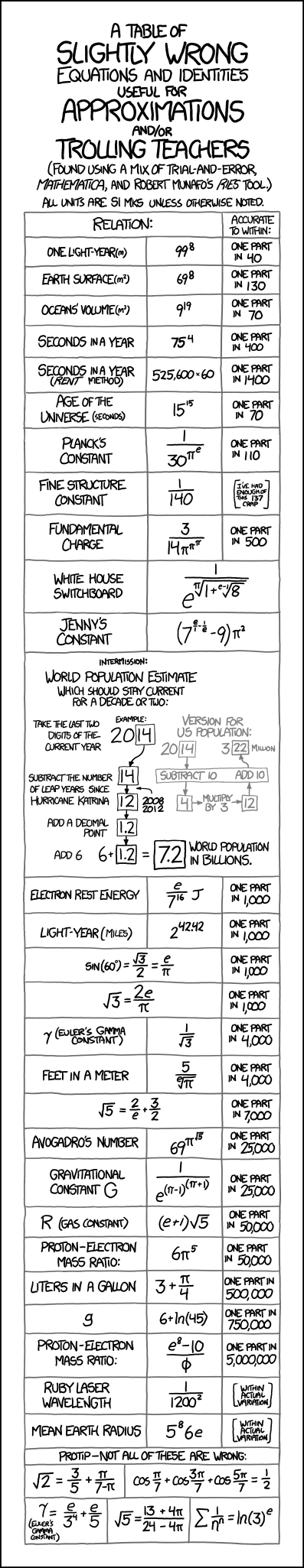← Back

April 25, 2012

# #1047: ApproximationsA table of slightly wrong equations and identities useful for approximations and

or trolling teachers. (Found using a mix of trial-and-error, Mathematica, and Robert Munafo’s Ries tool.) All units are SI MKS unless otherwise noted.

Relation:

One light year(m) ~=

99^8

Accurate to within:

one part in 40

Relation:

Earth Surface(m^2) ~=

69^8

Accurate to within:

one part in 130

Relation:

Ocean’s volume(m^3) ~=

9^19

Accurate to within:

one part in 70

Relation:

Seconds in a year ~=

75^4

Accurate to within:

one part in 400

Relation:

Seconds in a year (rent method) ~=

525,600 x 60

Accurate to within:

one part in 1400

Relation:

Age of the universe (seconds) ~=

15^15

Accurate to within:

one part in 70

Relation:

Planck’s constant ~=

1

(30^pi^e)

Accurate to within:

one part in 110

Relation:

Fine structure constant ~=

1

140

Accurate to within:

[I’ve had enough of this 137 crap]

Relation:

Fundamental charge ~=

3

(14 * pi^pi^pi)

Accurate to within:

one part in 500

Relation:

White House Switchboard ~=

1

(e^((1+(8)^(1

(e-1))^(1

pi))

Relation:

Jenny’s Constant ~=

(7^(e

1- 1

e) - 9) * pi^2

Intermission: World Population Estimate which should stay current for a decade or two:

Take the last two digits of the current year

Example: 20

Subtract the number of leap years since hurricane Katrina

Example:14 (minus 2008 and 2012) is 12

Add a decimal point

Example: 1.2

Example: 6 + 1.2

7.2 ~= World population in billions.

Version for US population:

Example: 20

Subtract 10

Example: 4

Multiply by 3

Example: 12

Example: 3 million

Relation:

Electron rest energy ~=

e

7^16 Joules

Accurate to within:

one part in 1000

Relation:

Light-year(miles) ~=

2^42.42

Accurate to within:

one part in 1000

Relation:

sin(60 degrees) = (3^(1

2))

2 ~=

e

pi

Accurate to within:

one part in 1000

Relation:

(3)^(1

1. ~=

2e

pi

Accurate to within:

one part in 1000

Relation:

gamma(Euler’s gamma constant) ~=

1

(3^(1

2))

Accurate to within:

One part in 4000

Relation:

Feet in a meter ~=

5

(pi^(1

e))

Accurate to within:

one part in 4000

Relation:

(5)^(1

1. ~=

2

e + 3

2

Accurate to within:

one part in 7000

Relation:

69^pi^5^(1

Accurate to within:

one part in 25,000

Relation:

R(gas constant) ~=

(e+1) * (5^(1

Accurate to within:

one part in 50,000

Relation:

Proton-electron mass ratio ~=

6*pi^5

Accurate to within:

one part in 50,000

Relation:

Liters in a gallon ~=

3+pi

4

Accurate to within:

one part in 500,000

Relation:

g ~=

6+ln(45)

Accurate to within:

one part in 750,000

Relation:

Proton-electron mass ratio ~=

(e^8 -10)

phi

Accurate to within:

one part in 5,000,000

Relation:

Ruby laser wavelength ~=

1

1200^2

Accurate to within:

[within actual variation]

Relation:

Mean Earth Radius ~=

(5^8)*6e

Accurate to within:

[within actual variation]

Protip - not all of these are wrong:

2^(1

1. ~=

3

5+pi

(7-pi)

cos(pi

• cos(3pi
• cos(5pi
1. ~=

1

2

gamma(Euler’s gamma constant) ~=

e

3^4 + e

5

5^(1

1. ~=

(13 + 4pi)

(24 - 4pi)

sigma(1

n^n) ~=

ln(3)^e